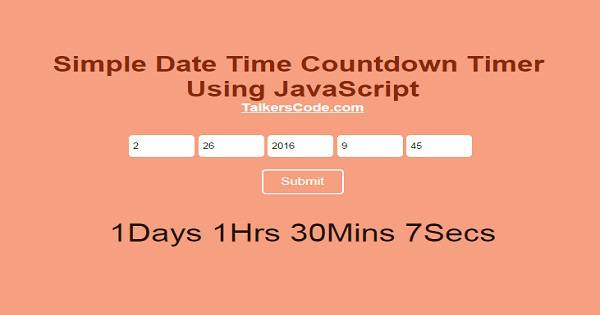# Simple Date Time Countdown Timer Using JavaScript

Last Updated : Apr 15, 2022

IN - JavaScript HTML

Countdown Timer is a great way to display the remaining time to a particular event.It creates a sense of excitement among the users and then they continuously visit for the event.

In this tutorial we will create a simple and cool Countdown Timer Using JavaScript.You may also like Value Countdown Using jQuery## To Create Date Time Countdown Timer it takes only two steps:-

1. Make a HTML file and define markup for countdown
2. Make a JavaScript file and define script for countdown

### Step 1. Make a HTML file and define markup for countdown

We make a HTML file and save it with a name countdown.html

```<html>
<script type="text/javascript" src="countdown.js"></script>
<body>

<input type="text" id="month" placeholder="Month">
<input type="text" id="day" placeholder="Day">
<input type="text" id="year" placeholder="Year">
<input type="text" id="hour" placeholder="Hour">
<input type="text" id="min" placeholder="Min">
<input type="button" value="Submit" onclick="settimer();">
<p id="timer_value"></p>

</body>
</html>
```

In this step we made 5 text box for user to enter date and time for countdown and click the submit button to start the countdown.

We include countdown.js which we will made in next step.You may also like session timeout warning with countdown using PHP and jQuery.

### Step 2. Make a JavaScript file and define script for countdown

We make a JavaScript file and save it with name countdown.js

```var timer;
function settimer()
{
clearInterval(timer);

var timer_month=document.getElementById("month").value;
var timer_day=document.getElementById("day").value;
var timer_year=document.getElementById("year").value;
var timer_hour=document.getElementById("hour").value;
if(timer_hour=="")timer_hour=0;
var timer_min=document.getElementById("min").value;
if(timer_min=="")timer_min=0;

var timer_date=timer_month+"/"+timer_day+"/"+timer_year+" "+timer_hour+":"+timer_min;
var end = new Date(timer_date); // Arrange values in Date Time Format
var now = new Date(); // Get Current date time
var second = 1000; // Total Millisecond In One Sec
var minute = second * 60; // Total Sec In One Min
var hour = minute * 60; // Total Min In One Hour
var day = hour * 24; // Total Hour In One Day

function showtimer() {
var now = new Date();
var remain = end - now; // Get The Difference Between Current and entered date time
if(remain < 0)
{
clearInterval(timer);
document.getElementById("timer_value").innerHTML = 'Reached!';
return;
}
var days = Math.floor(remain / day); // Get Remaining Days
var hours = Math.floor((remain % day) / hour); // Get Remaining Hours
var minutes = Math.floor((remain % hour) / minute); // Get Remaining Min
var seconds = Math.floor((remain % minute) / second); // Get Remaining Sec

document.getElementById("timer_value").innerHTML = days + 'Days ';
document.getElementById("timer_value").innerHTML += hours + 'Hrs ';
document.getElementById("timer_value").innerHTML += minutes + 'Mins ';
document.getElementById("timer_value").innerHTML += seconds + 'Secs';
}
timer = setInterval(showtimer, 1000); // Display Timer In Every 1 Sec
}
```

In this step we create a function to set and display the remaining time in every 1 sec.In this function first we clear all the interval in case any found will be stopped to stop the function collision.

Then we get the user entered value and current date time and then we get the difference and if the difference is 0 or less than 0 then it prints Reached! then we use Math.floor() function to get Date Time and display in every 1 sec using setInterval function you can set any time in this function.

There is also a Digital Clock Using CSS And JavaScript tutorial have a look.

Thats all, this is how to Create Simple Date Time Countdown Timer Using JavaScript. You can customize this code further as per your requirement. And please feel free to give comments on this tutorial.

Latest Tutorials